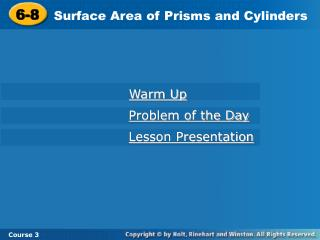DownloadDownload Presentation6-8

# 6-8

Télécharger la présentation## 6-8

- - - - - - - - - - - - - - - - - - - - - - - - - - - E N D - - - - - - - - - - - - - - - - - - - - - - - - - - -
##### Presentation Transcript

1. 6-8 Surface Area of Prisms and Cylinders Warm Up Problem of the Day Lesson Presentation Course 3

2. 6-8 Surface Area of Prisms and Cylinders Course 3 Warm Up 1. A triangular pyramid has a base area of 1.2 m2 and a height of 7.5 m. What is the volume of the pyramid? 2. A cone has a radius of 4 cm and a height of 10 cm. What is the volume of the cone to the nearest cubic centimeter?Use 3.14 for p. 3 m3 167 cm3

3. 6-8 Surface Area of Prisms and Cylinders 1 8 Course 3 Problem of the Day An ice cream cone is filled halfway to the top. The radius of the filled part is half the radius at the top. What fraction of the cone’s volume is filled?

4. 6-8 Surface Area of Prisms and Cylinders Course 3 Learn to find the surface area of prisms and cylinders.

5. 6-8 Surface Area of Prisms and Cylinders Course 3 Insert Lesson Title Here Vocabulary surface area lateral face lateral surface

6. 6-8 Surface Area of Prisms and Cylinders Course 3 Surface area is the sum of the areas of all surfaces of a figure. The lateral faces of a prism are parallelograms that connect the bases. The lateral surface of a cylinder is the curved surface.

7. 6-8 Surface Area of Prisms and Cylinders Course 3

8. 6-8 Surface Area of Prisms and Cylinders 1 2 = 2( • 8 • 3) + (18)(10) Course 3 Additional Example 1: Finding Surface Area Find the surface area of each figure A. S = 2pr2 + 2prh = 2p(42) + 2p(4)(6) = 80p in2 251.2 in2 B. S = 2B + Ph = 204 ft2

9. 6-8 Surface Area of Prisms and Cylinders = 2( • 7 • 6) + (21)(10) 1 2 Course 3 Try This: Example 1 Find the surface area of each figure 15 cm A. S = 2pr2 + 2prh 3 cm = 2p(152) + 2p(15)(3) = 540p in2 1695.6 cm2 7 cm 7 cm 6 cm B. S = 2B + Ph 10 cm 7 cm = 252 cm2

10. 6-8 Surface Area of Prisms and Cylinders Course 3 Additional Example 2: Exploring the Effects of Changing Dimensions A cylinder has diameter 8 in. and height 3 in. Explain whether tripling the height would have the same effect on the surface area as tripling the radius. They would not have the same effect. Tripling the radius would increase the surface area more than tripling the height.

11. 6-8 Surface Area of Prisms and Cylinders Course 3 Try This: Example 2 A cylinder has diameter 6 in. and height 2 in. Explain whether doubling the height would have the same effect on the surface area as doubling the radius. S = 2pr2 + 2pr(2h) S = 2pr2 + 2p(2r)h S = 2pr² + 2prh = 2p(3)2 + 2p(3)(4) = 2p(6) 2 + 2p(3)(2) = 2p(3)2 + 2p(3)(2) = 42p in2≈ 131.88 in2 = 84p in2≈ 263.76 in2 = 30p in2 ≈ 94.2 in2 They would not have the same effect. Doubling the radius would increase the surface area more than doubling the height.

12. 6-8 Surface Area of Prisms and Cylinders Course 3 Additional Example 3: Application A cylindrical soup can is 7.6 cm in diameter and 11.2 cm tall. What is the area of the label that covers the side of the can? Only the lateral surface needs to be covered. L = 2rh = 2(3.8)(11.2) The diameter is 7.6 cm, so r = 3.8 cm. ≈ 267.3 cm2

13. 6-8 Surface Area of Prisms and Cylinders Course 3 Try This: Example 3 A cylindrical storage tank that is 6 ft in diameter and 12 ft tall needs to be painted. The paint will cover 100 square feet per gallon. How many gallons will it take to paint the tank? S = 2r2 + 2rh The diameter is 6 ft, so r = 3 ft. = 2(32) + 2(3)(12) ≈ 282.6 ft2 Move the decimal point 2 places to the left to divide by 100. ≈ 2.826 gal

14. 6-8 Surface Area of Prisms and Cylinders Course 3 Insert Lesson Title Here Lesson Quiz Find the surface area of each figure to the nearest tenth. Use 3.14 for p. 1. the triangular prism 2. the cylinder 360 cm2 320.3 in2 3. All outer surfaces of a box are covered with gold foil, except the bottom. The box measures 6 in. long, 4 in. wide, and 3 in. high. How much gold foil was used? 84 in2